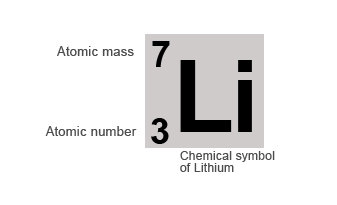Radioactivity is the process in which unstable atomic nuclei spontaneously decompose to form nuclei with a higher stability by the release of energetic sub atomic particles.

In order to understand radioactivity the structure of atoms needs to be understood.

## Atomic Structure

Atoms are made up of protons, neutrons and electrons each with their own properties.

• Protons have a positive (+) charge
• Electrons have a negative (-) charge
• Neutrons have no charge (Neutral)

The protons and neutrons lie in the nucleus at the centre of the atom and the electrons orbit round the nucleus. The proton and neutron are approximately equal in mass and they are each about 1800 times the mass of an electron.

The Hydrogen (H) Atom

The hydrogen atom is the simplest atom. It consists of a nucleus containing a positively charge proton around which a single negatively charged electron orbits.

Both charged particles in the atom are opposite and equal and so the atom is neutral. If the hydrogen atom loses the electron, it no longer remains neutral and the proton in the nucleus gives it an overall positive charge. In this case it is called a positively charged ion, H+

The Helium (He) Atom

The Helium atom contains two positively charge protons and 2 uncharged neutrons in the nucleus. It contains two negatively charged electrons in orbit round the nucleus to balance the positively charged protons.

## Atomic Number and Atomic Mass

The atomic number of an element is the number of protons that lie in the nucleus of an atom.

The atomic mass or mass number of an element is the total number of protons and neutrons that lie in the nucleus of an atom.

### Example:

If we take the example of the Lithium atom it contains 3 protons and 4 neutrons in its nucleus.

Therefore it's:

Atomic number = number of protons = 3
Mass number = number of protons + number of neutron = 3 + 4 = 7

Elements are normally represented by their chemical symbols along with their atomic and mass number, as shown below: